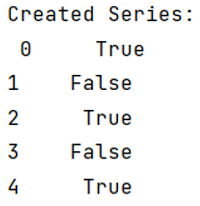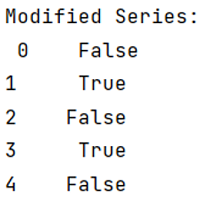# How to obtain the element-wise logical NOT of a Pandas Series?

Given a pandas series, we need to find element wise NOT on this series. By Pranit Sharma Last updated : September 20, 2023

Columns are the different fields that contain their particular values when we create a DataFrame. We can perform certain operations on both rows & column values. Pandas Series is just like columns. It is a One-dimensional array that contains a heterogeneous type of data.

## Problem statement

Given a pandas series, we need to find element wise NOT on this series.

## Obtaining the element-wise logical NOT of a Pandas Series

There is a certain way to initiate a pandas series, the simplest way is to define an array and pass it inside pandas.Series() method. This method of Pandas allows us to create a Series. The pandas.Series() method takes an array as a parameter.

Syntax:

```class pandas.Series(
data=None,
index=None,
dtype=None,
name=None,
copy=False,
fastpath=False
)
```
Note

To work with pandas, we need to import pandas package first, below is the syntax:

```import pandas as pd
```

Let us understand with the help of an example.

## Create and print a Pandas Series

```# Importing Pandas package
import pandas as pd

# Creating an array of elements
# containing Boolean values
arr = [True, False, True, False, True]

# Creating a Series
ser = pd.Series(arr)

# Display Series
print("Created Series:\n",ser)
```

### Output

The output of the above program is:Now, we are going to find element-wise NOT on this Series, for this purpose we are going to use "not" keyword.

## Python program to obtain the element-wise logical NOT of a Pandas Series

```# Creating an empty array
emp = []

# Traversing the Series and appending the NOT of
# each element of Series in empty array
for i in ser:
emp.append(not(i))

# Updating series by converting empty array
# again into Series
ser = pd.Series(emp)

# Display modified Series
print("Modified Series:\n",ser)
```

### Output

The output of the above program is: